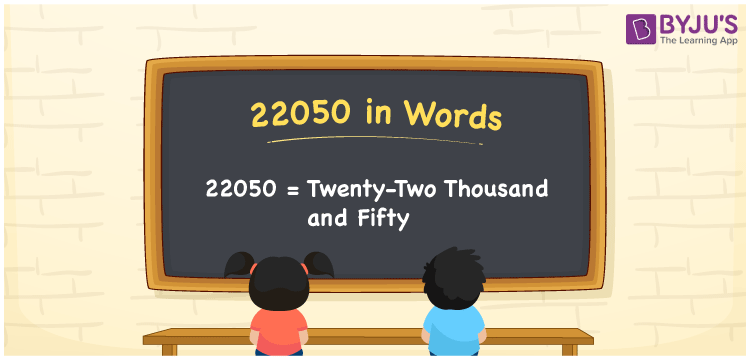# 22050 in Words

22050 in words is written as Twenty-two thousand and fifty. Suppose a journal contains 22050 entries; we can say, “The journal has Twenty-two thousand and fifty entries in it”. Here, Twenty-two thousand and fifty is the number name for 22050. In this article, you will understand how to write the cardinal number 22050 in words with the help of a place value chart.

 22050 in words Twenty-two thousand and fifty Twenty-two thousand and fifty in Numbers 22050

## 22050 in English words

Generally, we write numbers in words using the English alphabet. So, we can spell 22050 in English as “Twenty-two thousand and fifty”.## How to Write 22050 in Words?

To convert the number 22050 to words, we need to make a five-column place value chart since 22050 has five digits. The below table shows the place values for all digits of 22050.

 Ten thousand Thousands Hundreds Tens Ones 2 2 0 5 0

Here, ones = 0, tens = 5, hundred = 0, thousands = 2, ten thousands = 2

By expanding the above digits, we get;

2 × Ten thousand + 2 × Thousand + 0 × Hundred + 5 × Ten + 0 × One

= 2 × 10000 + 2 × 1000 + 0 × 100 + 5 × 10 + 0 × 1

= 20000 + 2000 + 50

= Twenty thousand + Two thousand + Fifty

= Twenty-two thousand and fifty

Therefore, 22050 in words = Twenty-two thousand and fifty.

As we know, 22050 is a natural number that is the successor of 22049 and the predecessor of 22051.

22050 in words – Twenty-two thousand and fifty

Is 22050 an odd number? – No

Is 22050 an even number? – Yes

Is 22050 a perfect square number? – No

Is 22050 a perfect cube number? – No

Is 22050 a prime number? – No

Is 22050 a composite number? – Yes

## Frequently Asked Questions on 22050 in Words

Q1

### How do you write 22050 in words?

We can write the number 22050 in words as “Twenty-two thousand and fifty”.
Q2

### How to write Rs. 22050 in words on a cheque?

On a cheque, an amount of Rs. 22050 can be written in words as “Twenty-two thousand and fifty rupees only”.
Q3

### Express the answer for 22050 – 14050 in words.

22050 – 14050 = 8000 Thus, the value of 22050 – 14050, i.e., 8000 can be expressed in words as Eight thousand.